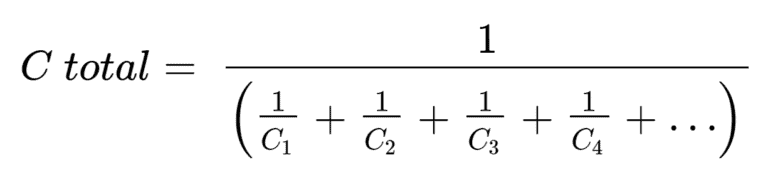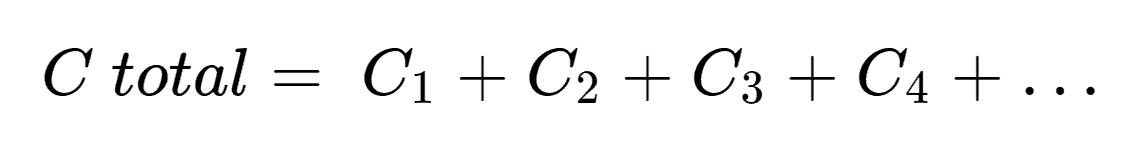# Capacitance Calculators

### Series Capacitor

C1:
C2:
C3:
C4:
C5:
C6:
C7:
C8:
C9:
C10:
Total Series Capacitance
=

### Parallel Capacitor

C1:
C2:
C3:
C4:
C5:
C6:
C7:
C8:
C9:
C10:
Total Parallel Capacitance
=

## Parallel Capacitance FormulaCapacitance is the ability of an object to store electric charges and it relies on the shape of the capacitor and the choice of the dielectric material used in between the plates. There are two types of calculations that can be done with capacitance. Series or parallel, which depends on the arrangement in the circuit.

## Series Capacitor

A series capacitor calculator will calculate the total series capacitance of a circuit. Which allows for up to ten different capacitor values. As you know, capacitors can be arranged in a circuit, when capacitors are combined in a series, the charge, in each of those are the same. They are the same because the fundamental property of capacitors is that the absolute value of the charge stored on both plates is the same but with the opposing charge.

A series capacitor calculator Suntsu has created, performs this calculation below accounting for up to ten values.## Parallel Capacitor

A parallel capacitor calculator will sum the estimated total parallel capacitance in a circuit. This calculator can sum up to ten different capacitor values. When capacitors are arranged in parallel in a system with voltage source, V, the voltages of each element are the same and are equal to the source capacitor. The reason for this is because the top plate of the source capacitor is connected to the top plate of the next capacitor, which is connected to the top plate of the next capacitor, and so on until you reach 10 capacitors. The same thing applies when they are connected by their bottom plates.

A parallel capacitor calculator Suntsu has created, performs this calculation below accounting for up to ten values.